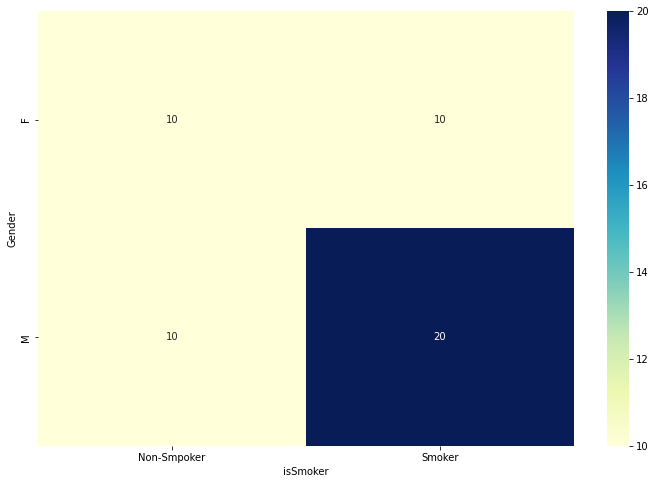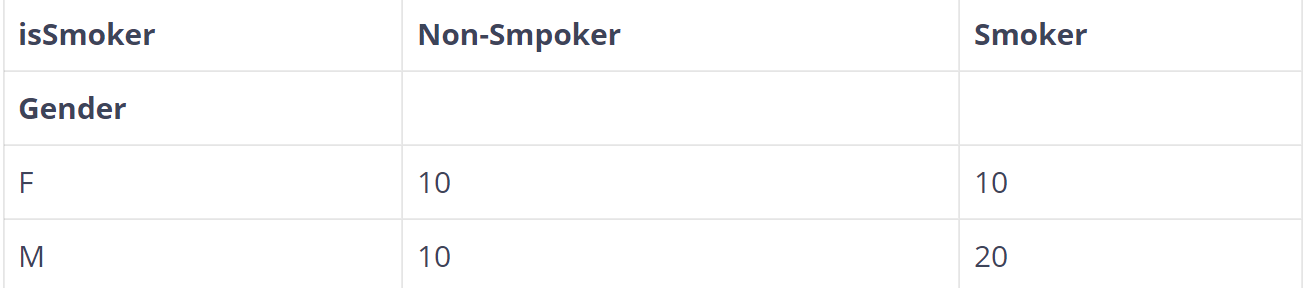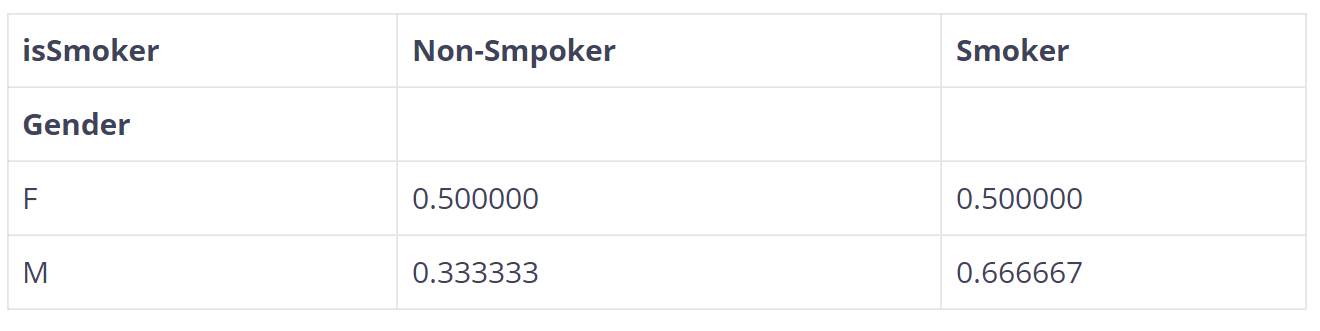# How to Run the Chi-Square Test in PythonExample of Chi-Square Test in Python. We will provide a practical example of how we can run a Chi-Square Test in Python.

We will provide a practical example of how we can run a Chi-Square Test in Python. Assume that we want to test if there is a statistically significant difference in Genders *(M, F) population between *Smokers *and *Non-Smokers. Let’s generate some sample data to work on it.

## Sample Data

``````mport pandas as pd
import numpy as np
from scipy.stats import chi2_contingency

import seaborn as sns
import matplotlib.pyplot as plt
%matplotlib inline
df = pd.DataFrame({'Gender' : ['M', 'M', 'M', 'F', 'F'] * 10,
'isSmoker' : ['Smoker', 'Smoker', 'Non-Smpoker', 'Non-Smpoker', 'Smoker'] * 10
})

Output:

``````  Gender isSmoker
0 M Smoker
1 M Smoker
2 M Non-Smpoker
3 F Non-Smpoker
4 F Smoker``````

## Contingency Table

To run the Chi-Square Test, the easiest way is to convert the data into a contingency table with frequencies. We will use the `crosstab` command from `pandas`.

``````contigency= pd.crosstab(df['Gender'], df['isSmoker'])
contigency``````Let’s say that we want to get the percentages by Gender (row)

``````contigency_pct = pd.crosstab(df['Gender'], df['isSmoker'], normalize='index')
contigency_pct``````If we want the percentages by column, then we should write normalize=’column’ and if we want the total percentage then we should write normalize=’all’

## top 30 Python Tips and Tricks for Beginners

In this post, we'll learn top 30 Python Tips and Tricks for Beginners